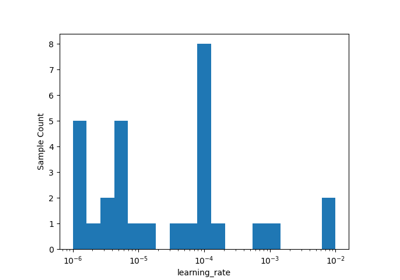# skopt.plots.plot_objective_2D¶

skopt.plots.plot_objective_2D(result, dimension_identifier1, dimension_identifier2, n_points=40, n_samples=250, levels=10, zscale='linear', sample_source='random', minimum='result', n_minimum_search=None, ax=None)[source][source]

Create and return a Matplotlib figure and axes with a landscape contour-plot of the last fitted model of the search-space, overlaid with all the samples from the optimization results, for the two given dimensions of the search-space.

This is similar to plot_objective() but only for 2 dimensions whose doc-string also has a more extensive explanation.

Parameters
resultOptimizeResult

The optimization results e.g. from calling gp_minimize().

dimension_identifier1str or int

Name or index of a dimension in the search-space.

dimension_identifier2str or int

Name or index of a dimension in the search-space.

n_samplesint, default=250

Number of random samples used for estimating the contour-plot of the objective function.

n_pointsint, default=40

Number of points along each dimension where the partial dependence is evaluated when generating the contour-plots.

levelsint, default=10

Number of levels to draw on the contour plot.

zscalestr, default=’linear’

Scale to use for the z axis of the contour plots. Either ‘log’ or linear for all other choices.

axMatplotlib.Axes, default: None

When set, everything is plotted inside this axis.

Returns
axMatplotlib.Axes

The Matplotlib Figure-object. For example, you can save the plot by calling fig.savefig('file.png')

## Examples using skopt.plots.plot_objective_2D¶